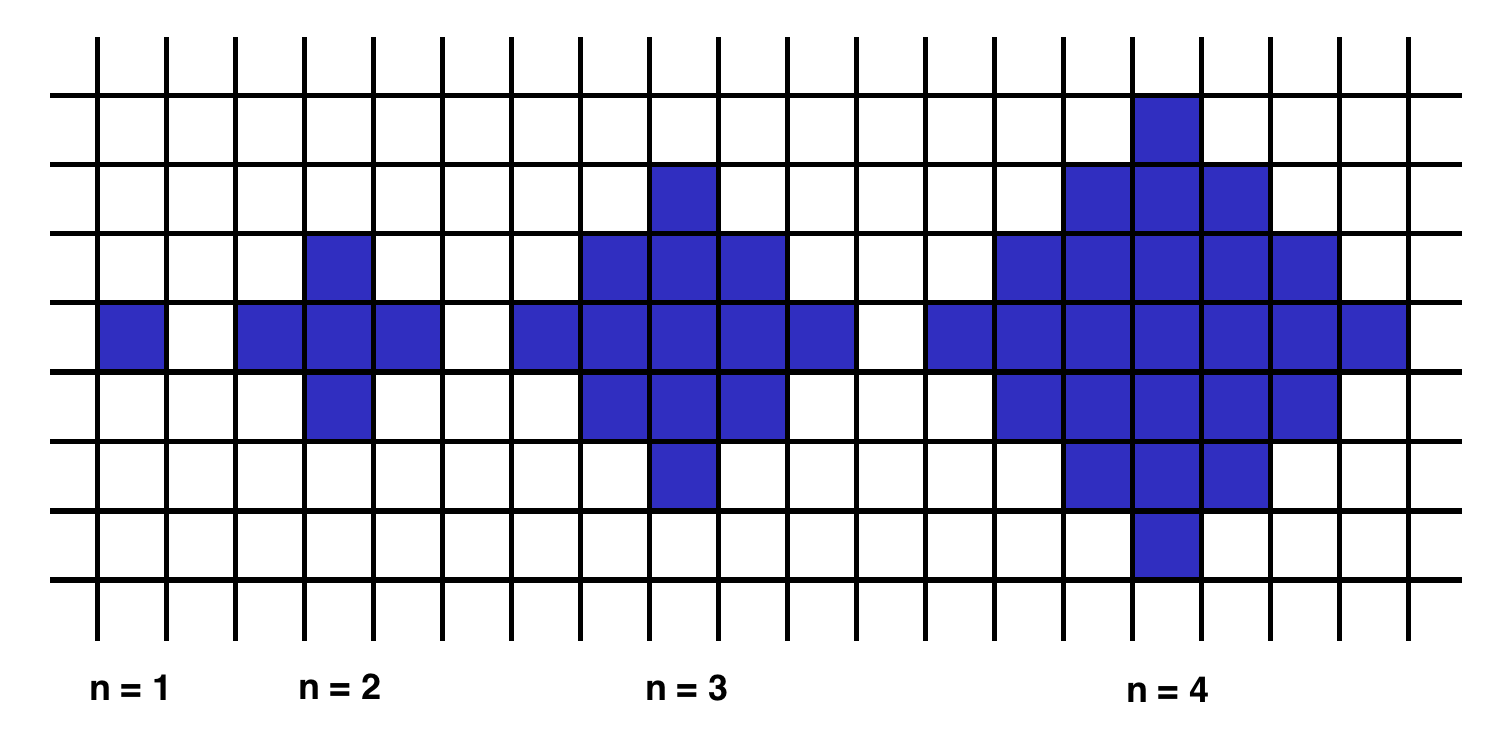# kentakang's blog

Computer/Algorithm

## Daily Algorithm - shapeArea

kentakang 2018. 1. 31. 02:30
반응형

Below we will define an `n`-interesting polygon. Your task is to find the area of a polygon for a given `n`.

A `1`-interesting polygon is just a square with a side of length `1`. An `n`-interesting polygon is obtained by taking the `n - 1`-interesting polygon and appending `1`-interesting polygons to its rim, side by side. You can see the `1`-, `2`-, `3`- and `4`-interesting polygons in the picture below.Example

• For `n = 2`, the output should be
`shapeArea(n) = 5`;
• For `n = 3`, the output should be
`shapeArea(n) = 13`.

Input/Output

• [execution time limit] 3 seconds (java)

• [input] integer n

Guaranteed constraints:
`1 ≤ n < 104`.

• [output] integer

The area of the `n`-interesting polygon.

Solution
``````public class ShapeArea {
static int shapeArea(int n) {
if(n == 1)
return 1;

return shapeArea(n - 1) + 4 * (n - 1);
}

public static void main(String[] args) {
System.out.println(shapeArea(2));
}
}
``````

반응형